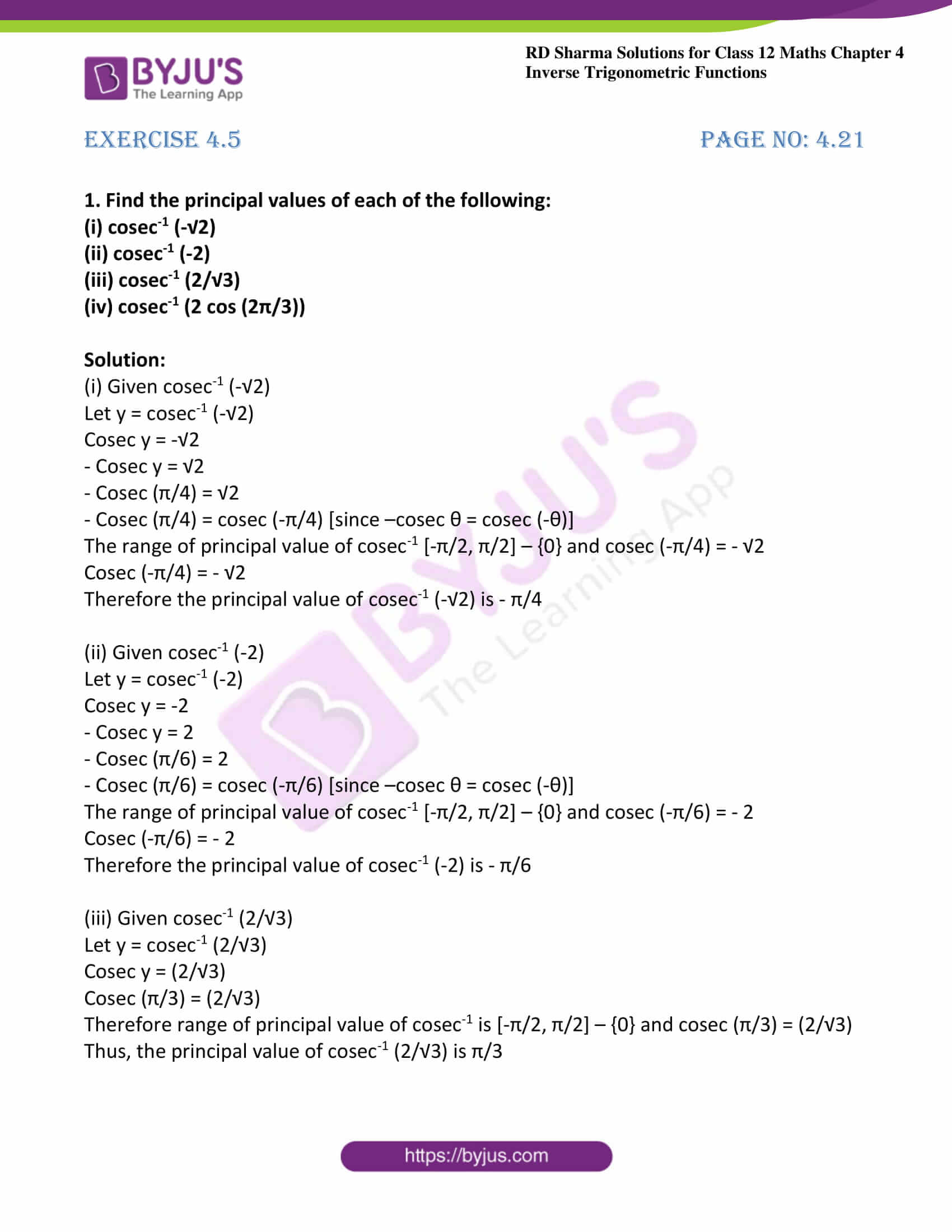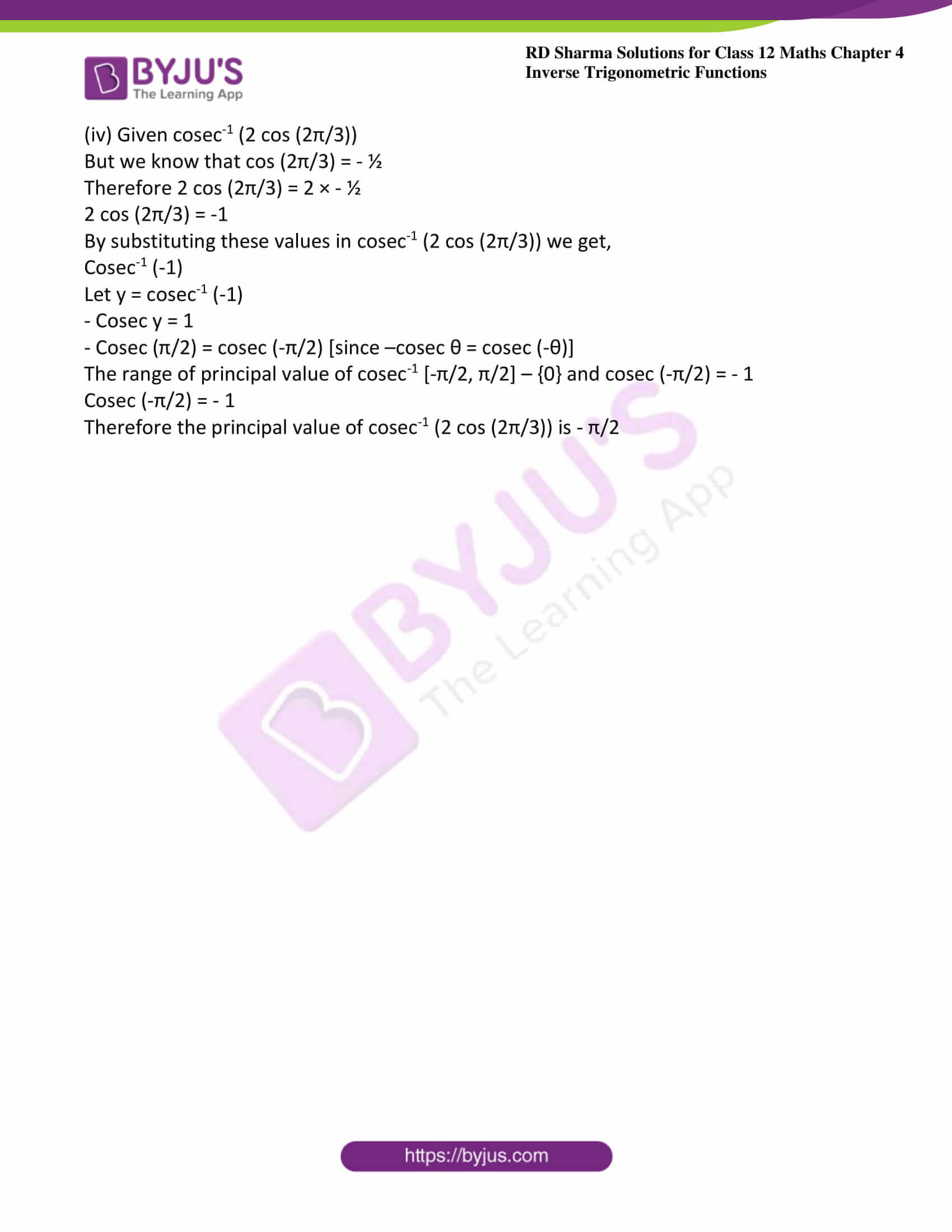# RD Sharma Solutions For Class 12 Maths Exercise 4.5 Chapter 4 Inverse Trigonometric Functions

RD Sharma Solutions Class 12 Maths Exercise 4.5 Chapter 4 Inverse Trigonometric Functions is provided here. Exercise 4.5 of Chapter 4 consists of problems based on inverse of cosecant function. Students who are unable to solve exercise wise problems as per RD Sharma textbook can make use of solutions designed by expert faculty at BYJU’S.

These RD Sharma Solutions are explained in the best possible way to provide a clear idea about the concepts among students. It mainly improves their speed in solving problems, which builds time management that is important from the exam point of view. To get doubts cleared instantly, students can download RD Sharma Solutions Class 12 Maths Chapter 4 Inverse Trigonometric Functions Exercise 4.5 PDF, which are provided here.

## Download the PDF of RD Sharma Solutions For Class 12 Chapter 4 – Inverse Trigonometric Functions Exercise 4.5### Exercise 4.5 Page No: 4.21

1. Find the principal values of each of the following:

(i) cosec-1 (-√2)

(ii) cosec-1 (-2)

(iii) cosec-1 (2/√3)

(iv) cosec-1 (2 cos (2π/3))

Solution:

(i) Given cosec-1 (-√2)

Let y = cosec-1 (-√2)

Cosec y = -√2

– Cosec y = √2

– Cosec (π/4) = √2

– Cosec (π/4) = cosec (-π/4) [since –cosec θ = cosec (-θ)]

The range of principal value of cosec-1 [-π/2, π/2] – {0} and cosec (-π/4) = – √2

Cosec (-π/4) = – √2

Therefore the principal value of cosec-1 (-√2) is – π/4

(ii) Given cosec-1 (-2)

Let y = cosec-1 (-2)

Cosec y = -2

– Cosec y = 2

– Cosec (π/6) = 2

– Cosec (π/6) = cosec (-π/6) [since –cosec θ = cosec (-θ)]

The range of principal value of cosec-1 [-π/2, π/2] – {0} and cosec (-π/6) = – 2

Cosec (-π/6) = – 2

Therefore the principal value of cosec-1 (-2) is – π/6

(iii) Given cosec-1 (2/√3)

Let y = cosec-1 (2/√3)

Cosec y = (2/√3)

Cosec (π/3) = (2/√3)

Therefore range of principal value of cosec-1 is [-π/2, π/2] – {0} and cosec (π/3) = (2/√3)

Thus, the principal value of cosec-1 (2/√3) is π/3

(iv) Given cosec-1 (2 cos (2π/3))

But we know that cos (2π/3) = – ½

Therefore 2 cos (2π/3) = 2 × – ½

2 cos (2π/3) = -1

By substituting these values in cosec-1 (2 cos (2π/3)) we get,

Cosec-1 (-1)

Let y = cosec-1 (-1)

– Cosec y = 1

– Cosec (π/2) = cosec (-π/2) [since –cosec θ = cosec (-θ)]

The range of principal value of cosec-1 [-π/2, π/2] – {0} and cosec (-π/2) = – 1

Cosec (-π/2) = – 1

Therefore the principal value of cosec-1 (2 cos (2π/3)) is – π/2

### Access other exercises of RD Sharma Solutions For Class 12 Chapter 4 – Inverse Trigonometric Functions

Exercise 4.1 Solutions

Exercise 4.2 Solutions

Exercise 4.3 Solutions

Exercise 4.4 Solutions

Exercise 4.6 Solutions

Exercise 4.7 Solutions

Exercise 4.8 Solutions

Exercise 4.9 Solutions

Exercise 4.10 Solutions

Exercise 4.11 Solutions

Exercise 4.12 Solutions

Exercise 4.13 Solutions

Exercise 4.14 Solutions